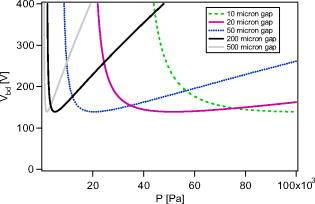# Minimum breakdown voltage in Neon, Electrode spacing in mm

• Shane Cox
In summary: The graph shows the electric field as a function of pressure at different electrode spacings. It is possible to calculate the electric field at any given pressure by plotting the voltage against the corresponding electrode spacing. The curve shown is for a given voltage, and corresponds to a given electrode spacing. So, if you want to know the electric field at 74762 microns of pressure, you would need to find the corresponding voltage on the graph, and then find the corresponding electrode spacing.In summary, the electric field at 74762 microns of pressure is higher when the voltage is higher, and the electrode spacing is ____.

#### Shane Cox

Hello all. I am conducting a simple research project, and am having a little trouble with the math for paschen's law.

my question is.

At 74762 Microns of pressure, using pure Neon gas, what is the electrode spacing in mm for the minimum breakdown voltage of that gas?

Thank you.

Welcome to the PF.Shane Cox said:
the minimum breakdown voltage of that gas?
The breakdown is based on the electric field. The electric field depends on the applied voltage and the electrode spacing. The minimum breakdown voltage is when the electrode spacing is ________. Can you fill in the blank?

I am unable to answer the question, as I have been unable to do the calculations correctly, they are beyond my current ability.

I do understand that neon has a minimum breakdown voltage, the voltage i will be running is 260V DC

which is a little above the minimum threshold, so the optimum gap distance for the pressure I specified is what I am unable to calculate.

The units of electric field are Volts per meter. So the electric field is higher when the voltage is higher, and the electrode spacing is ____ (maximum or minimum?)?This Graph shows the optimum pressure to electrode spacing for the minimum breakdown voltage of the gas used in this Graph, theelectrode spacing is what I am trying calculate for pure Neon at its minimum breakdown voltage at 74762 microns of pressure.

#### Attachments

Another way of explaining it is that, I am trying to apply the minimum amount of voltage in order to achieve breakdown in pure neon gas, at 74762 microns of pressure, and looking for the optimum electrode spacing in order to achieve this.

The Paschen Curve is for the breakdown electric field as a function of pressure. It may be labeled as "Voltage", but that will be for a given electrode spacing, giving a corresponding electric field. No?

## 1. What is the minimum breakdown voltage in neon?

The minimum breakdown voltage in neon is the minimum amount of voltage needed to cause a spark to form between two electrodes in a neon gas environment. This voltage can vary depending on factors such as gas pressure, electrode spacing, and gas purity.

## 2. How is the minimum breakdown voltage in neon determined?

The minimum breakdown voltage in neon is determined by conducting experiments in which the voltage is gradually increased until a spark is observed between the electrodes. This voltage is then recorded as the minimum breakdown voltage for that specific setup.

## 3. What is the significance of electrode spacing in determining the minimum breakdown voltage in neon?

Electrode spacing refers to the distance between the two electrodes in a neon gas environment. This distance plays a crucial role in determining the minimum breakdown voltage as it affects the electric field strength between the electrodes. A smaller electrode spacing will result in a higher electric field strength and thus a lower minimum breakdown voltage.

## 4. How does gas pressure affect the minimum breakdown voltage in neon?

Gas pressure is another important factor in determining the minimum breakdown voltage in neon. As the pressure of the gas increases, the distance between the gas molecules decreases, resulting in a higher number of collisions and a higher probability of ionization. This leads to a lower minimum breakdown voltage.

## 5. What are some practical applications of understanding the minimum breakdown voltage in neon?

Understanding the minimum breakdown voltage in neon is crucial in the design and operation of neon gas discharge devices, such as neon lights and neon signs. It also has applications in plasma physics research and in industrial processes that use neon gas as a dielectric medium. Additionally, knowledge of the minimum breakdown voltage in neon can help ensure the safe operation of high-voltage equipment.